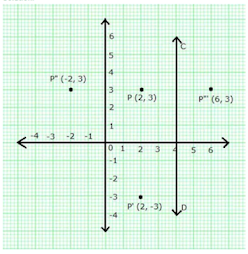# ICSE Solutions for Chapter 12 Reflection Class 10 Mathematics

Question 1: Attempt this question on graph paper.
(a) Plot A (3, 2) and B (5, 4) on graph paper. Take 2 cm = 1 unit on both the axes
(b) Reflect A and B in the x-axis to A’ and B’ respectively. Plot these points also on the same graph paper.
(c) Write down:
(i) the geometrical name of the figure ABB’A’;
(ii) the measure of angle ABB’;
(iii) the image of A” of A when A is reflected in the origin
(iv) the single transformation that maps A’ to A”

Solution 1:(c)
(i) From graph, it is clear that ABB’A” is an isosceles trapezium. The measure of angle ABB’ is 45˚
(iii) A” = (-3, -2)
(iv) Single transformation that maps A’ to A” is the reflection in y-axis.

Question 2: A point P is its own image under the reflection in a line L. Describe the position of point the P with respect to line l.

Solution 2:
Since, the point P is its own image under the reflection in the line l, So, point P is an invariant point.
Hence, the position of point P remains unaltered.

Question 3: (i) Point P (a, b) is reflected in the x-axis to P’ (5, -2). Write down the values of a and b.
(ii) P" is the image of P when reflected in the y-axis. Write down the co-ordinates of P”.
(iii) Name a single transformation that maps P' to P”.

Solution 3:
We know Mx (x, y) = (x, -y)
P’(5, -2) = reflection of P (a, b) in x-axis.
Thus, the co-ordinates of Pare (5, 2).
Hence, a = 5 and b = 2.

(ii) P” = image of P (5, 2) reflected in y-axis = (-5, 2)

(iii) Single transformation that maps P’ to P” is the reflection in origin.

Question 4: The point (-2, 0) on reflection in a line is mapped to (2, 0) and the point (5, -6) on reflection in the same line is mapped to (-5, -6).
(i) State the name of the mirror line and write its equation
(ii) State the co-ordinates of the image of (-8, -5) in the mirror line.

Solution 4:
(i) We know reflection of a point (x, y) in y-axis is (-x, y).
Hence, the point (-2, 0) when reflected in y-axis is mapped to (2, 0).
Thus, the mirror line is the y-axis and its equation is x = 0

(ii) Co-ordinates of the image of (-8, -5) in the mirror line (i.e., y-axis) are (8, -5).

Question 5: State the co-ordinates of the following points under reflection in origin:
(i) (-2, -4)
(ii) (-2, 7)
(iii) (0, 0)

Solution 5: (i) (-2, -4)
The co-ordinate of the given point under reflection in origin is (2, 4).

(ii) (-2, 7)
The co-ordinate of the given point under reflection in origin is (2, -7).

(iii) (0, 0)
The co-ordinate of the given point under reflection in origin is (0, 0).

Question 6: State the co-ordinates of the following points under reflection in the line x = 0;
(i) (-6, 4)
(ii) (0, 5)
(iii) (3, -4)

Solution 6: (i) (-6, 4)
The co-ordinate of the given point under reflection in the line x = 0 is (6, 4).

(ii) (0, 5)
The co-ordinate of the given point under reflection in the line x = 0 is (0, 5).

(iii) (3, -4)
The co-ordinate of the given point under reflection in the line x = 0 is (-3, -4).

Question 7: A point P (a, b) is reflected in the x-axis to P’ (2, -3). Write down the values of a and b. P” is the image of P. reflected in the y-axis. Write down the co-ordinates of P”. Find the co-ordinates of P", when P is reflected in the line parallel to y-axis, such that x = 4.

Solution 7:A point P (a, b) is reflected in the x-axis to P’(2, -3).
We know Mx (x, y) = (x, -y)
Thus, co-ordinates of P are (2, 3). Hence, a = 2 and b = 3.
P’ = Image of reflected in the y-axis = (-2, 3)
P” = Reflection of Pin the line (x = 4) = (6, 3)

Question 8: A point P is reflected in the x-axis. Co-ordinates of its image are (-4, 5)
(i) Find the co-ordinates of P.
(ii) Find the co-ordinates of the image of P under reflection in the y-axis.

Solution 8:
(i) Since, Mx  (-4, -5) = (-4, 5)
So, the co-ordinates of P are (-4, -5).

(ii) Co-ordinates of the image of P under reflection in the y-axis (4, -5).

Question 9: A point P is reflected in the origin. Co-ordinates of its image are (-2, 7).
(i) Find the co-ordinates of P.
(ii) Find the co-ordinates of the image of P under reflection in the x-axis.

Solution 9:
Since, Mo (2, -7) = (-2, 7)
So, the co-ordinates of P are (2, -7).

(ii) Co-ordinates of the image of P under reflection in the x-axis (2, 7).

Question 10: The point P (5, 3) was reflected in the origin to get the image P'.
(a) Write down the co-ordinates of P’.
(b) If M is the foot if the perpendicular from P to the x-axis, find the co-ordinates of M.
(c) If N is the foot if the perpendicular from P’ to the x-axis, find the co-ordinates of N.
(d) Name the figure PMP’N.
(e) Find the area of the figure PMP’N.

Solution 10:(a) Co-ordinates of P’ = (-5,-3)
(b) Co-ordinates of M = (5, 0)
(c) Co-ordinates of N = (-5, 0)
(d) PMPN is a parallelogram.
(e) Area of PMP’N = 2 (Area of D PMN)
= 2 × ½  ×10 ×3
= 30 sq units